Next: Exercises Up: Non-Cartesian Coordinates Previous: Cylindrical Coordinates

# Spherical Coordinates

In the spherical coordinate system,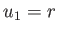,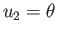, and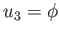, where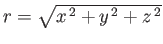,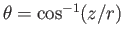,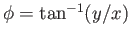, and,,are standard Cartesian coordinates. Thus,is the length of the radius vector,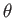the angle subtended between the radius vector and the-axis, and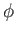the angle subtended between the projection of the radius vector onto the-plane and the-axis. (See Figure C.2.)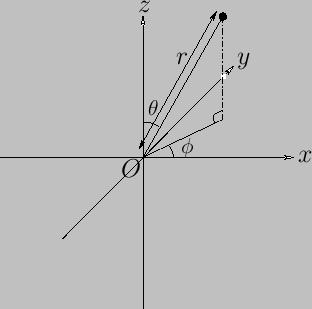A general vector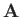is written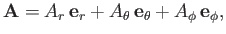(C.55)

where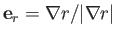,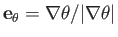, and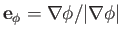. Of course, the unit vectors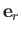,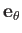, and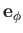are mutually orthogonal, so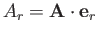, et cetera.

As is easily demonstrated, an element of length (squared) in the spherical coordinate system takes the form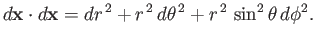(C.56)

Hence, comparison with Equation (C.6) reveals that the scale factors for this system are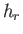(C.57)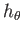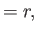(C.58)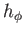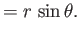(C.59)

Thus, surface elements normal to,, andare written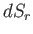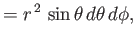(C.60)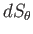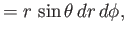(C.61)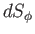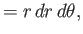(C.62)

respectively, whereas a volume element takes the form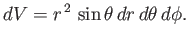(C.63)

According to Equations (C.13), (C.15), and (C.18), gradient, divergence, and curl in the spherical coordinate system are written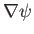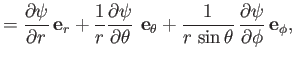(C.64)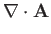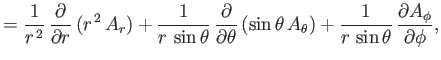(C.65)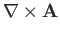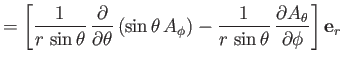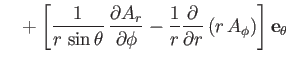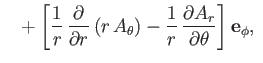(C.66)

respectively. Here,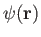is a general scalar field, anda general vector field.

According to Equation (C.19), when expressed in spherical coordinates, the Laplacian of a scalar field becomes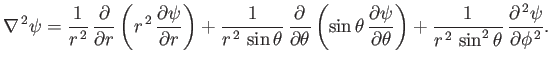(C.67)

Moreover, from Equation (C.23), the components of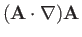in the spherical coordinate system are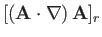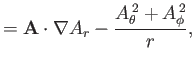(C.68)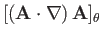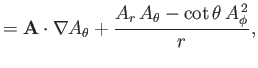(C.69)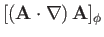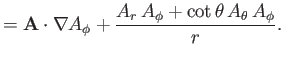(C.70)

Now, according to Equation (C.26), the components of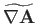in the spherical coordinate system are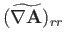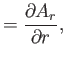(C.71)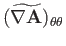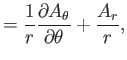(C.72)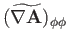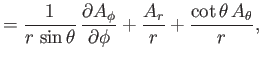(C.73)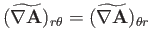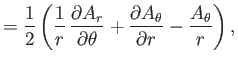(C.74)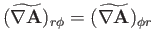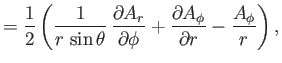(C.75)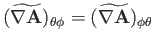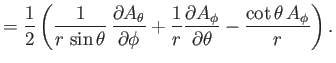(C.76)

Finally, from Equation (C.28), the components of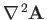in the spherical coordinate system are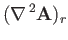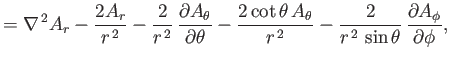(C.77)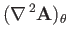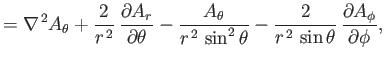(C.78)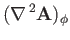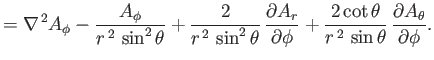(C.79)Next: Exercises Up: Non-Cartesian Coordinates Previous: Cylindrical Coordinates
Richard Fitzpatrick 2016-03-31